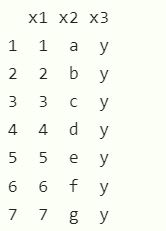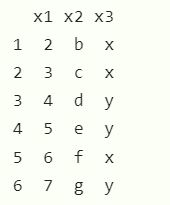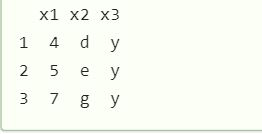# How to find common rows and columns between two dataframe in R?

• Last Updated : 26 Mar, 2021

Two data frames can have similar rows, and they can be determined. In this article, we will find the common rows and common columns between two data frames, in the R programming language.

Approach

• Create a first data frame
• Create a second data frame
• Compare using required functions
• Copy same rows to another data frame
• Display data frame so generated.

Data frames in use:

data1:data 2:Method 1: Using Intersect() Function:

Syntax: intersect(data , data2)

Parameters:

• data/data2 : It is the data frames on which we have to apply the function.

Example:

## R

 `data1 <- ``data.frame``(x1 = 1:7,                       ` `                    ``x2 = ``letters``[1:7], ` `                    ``x3 = ``"y"``) ` `data1  ` `data2 <- ``data.frame``(x1 = 2:7,                      ` `                   ``x2 = ``letters``[2:7], ` `                   ``x3 = ``c``(``"x"``, ``"x"``, ``"y"``, ``"y"` `, ``"x"``, ``"y"``)) ` `data2 ` ` `  `common_rows <- generics::``intersect``(data1, data2)   ` `common_rows`

Output:Method 2: Using inner_join() function.

To find the common data using this method first install the “dplyr” package in the R environment.

install.packages(“dplyr”)

This module has an inner_join() which finds inner join between two data sets.

Syntax: inner_join(data1,data2)

Parameter:

• data1/data2: two datasets to be compared

Example:

## R

 `library``(``"dplyr"``) ` ` `  `data1 <- ``data.frame``(x1 = 1:7,                       ` `                    ``x2 = ``letters``[1:7], ` `                    ``x3 = ``"y"``) ` `data1  ` `data2 <- ``data.frame``(x1 = 2:7,                      ` `                   ``x2 = ``letters``[2:7], ` `                   ``x3 = ``c``(``"x"``, ``"x"``, ``"y"``, ``"y"` `, ``"x"``, ``"y"``)) ` `data2    ` `common_rows2 <- ``inner_join``(data1, data2)           ` `common_rows2`

Output:My Personal Notes arrow_drop_up
Recommended Articles
Page :